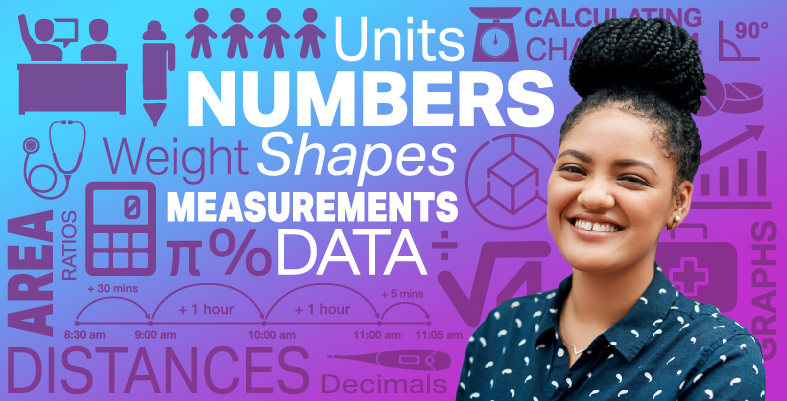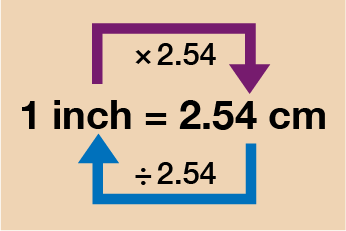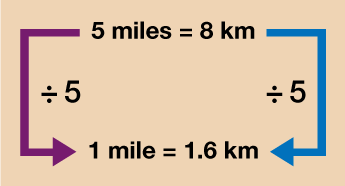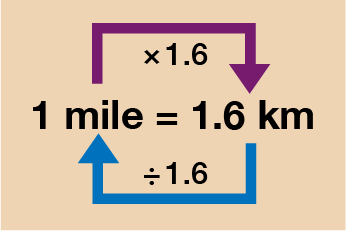Everyday maths for Health and Social Care and Education Support 2

Start this free course now. Just create an account and sign in. Enrol and complete the course for a free statement of participation or digital badge if available.

Free course

# 2.2 Converting units of measure between different systems

This is a useful skill to have because you may often measure something in one unit, say your height in feet and inches, but then need to convert it to centimetres. You may, for example, be training to run a 10 km race and want to know how many miles that is. To do this all you need to know is the conversion rate and then you can use your multiplication and division skills to calculate the answer.

When performing these conversions, you are converting between metric and imperial measures. Metric measures are commonly used around the world but there are some countries (the USA for example) who still use imperial units. Whilst the UK uses metric units (g, kg, m, cm etc.) there are some instances where you may still need to convert a metric measure to an imperial one (inches, feet, gallons etc.).

In most cases, the conversion rate will be given in a similar format to the way money exchange rates are given, i.e. 1 inch = 2.5 cm or 1 kg = 2.2 lb. In these cases you can do exactly the same as you would with a currency conversion. The only exceptions here are where you don’t have one of the units of measure as a single unit i.e. 5 miles = 8 km.

As this skill is very similar to the one you previously learnt with currency conversions, let’s just look at two brief examples before you have a go at an activity for yourself.

## Example: Centimetres and inches

You are running a craft activity with the children on a hospital ward. For the activity, each child needs a piece of material that is 15 inches wide. The material you have bought is 362 cm wide.

How many 15 inches pieces can you cut from the material you bought?

Use 1 inch = 2.54 cm.

Method

You need to start by converting either cm to inches or inches to cm so that you are working with units in the same system. Let’s look at both ways so that you feel confident that you will get the same answer either way.Figure 8 Converting between inches and centimetres

You can see from the diagram above that to convert from inches to cm you must multiply by 2.54. To convert from cm to inches you must divide by 2.54.

Converting inches to cm then:

15 inches × 2.54 = 38.1 cm. This is the length of the material needed for each child.

To calculate how many pieces of material you can cut you then do:

362 ÷ 38.1 = 9.5013…. As you need whole pieces of material, you need to round this answer down to 9 pieces.

Converting from cm to inches:

362 ÷ 2.54 = 142.5196…, this is the length of the big piece of material.

To calculate how many pieces of material you can cut you then do:

142.6196… ÷ 15 = 9.5013…. Again, as you need whole pieces of material, you need to round this answer down to 9 pieces.

You can see that no matter which way you choose to convert you will arrive at the same answer.

## Example: Kilometres and miles

You have signed up for a 60 km charity bike ride to raise money for the hospice you work at. There is a lake in a nearby park that you want to use for your training. You know that one lap of the lake is 2 miles. You want to cycle a distance of at least 40 km in your last training session before the race.

How many full laps of the lake should you do?

You know that a rough conversion is 5 miles = 8 km.

There is more than one way to go about this calculation and, as ever, if you have a different method that works for you and you arrive at the same answer, feel free to use it!

Method

Since you already know how to convert if you are given a conversion that has a single unit of whichever measure you are using, it makes sense to just change the given conversion into one that you are used to dealing with.

Look at the diagram below – since you know that 5 miles is worth 8 km, you can find out what 1 mile is worth by simply dividing 5 by 5 so that you arrive at 1 mile. Whatever you do to one side, you must do to the other. Therefore, you also do 8 divided by 5. This then gives 1 mile = 1.6 km.Figure 9 Calculating 1 mile in kilometres

Now that you know 1 mile = 1.6 km, you can solve this problem in its usual way:Figure 10 Converting between miles and kilometres

Since you first want to know what 40 km is in miles you need to divide by 1.6:

• 40 ÷ 1.6 = 25 miles

As each lap of the lake is 2 miles you then need to divide 25 miles by 2 to find the total number of laps you need to complete:

• 25 ÷ 2 = 12.5 laps

Therefore, you will need to cycle 12.5 laps around the lake in order to have cycled your target of 40 km.

Now that you have seen a couple of worked examples, have a go at the activity below to check your understanding.

## Activity 4: Converting between systems

1. You need to buy a storage unit for the children’s centre you work at. The space that the unit needs to fit in is 185 cm wide. The storage unit you want to purchase is 6 feet wide.

Will the unit fit into the space?

Use 1 foot = 30.48 cm.

2. The school you work at has run out of milk and you have been asked to go to the shop and buy 10 pints. When you arrive however, the milk is only available in 2 litre bottles. You know that 1 litre = 1.75 pints. How many 2 litre bottles should you buy?

3. You are packing to go on holiday and are allowed 25 kg of luggage on the flight. You’ve weighed your case on the bathroom scales and it weighs 3 stone and 3 pounds.

You know that:

• 1 stone = 14 pounds

2.2 pounds = 1 kg

Is your luggage over the weight limit?

1. As you want to convert from cm to feet you do:

• 185 ÷ 30.48 = 6.07 to two d.p.

As the space is 6.07 feet wide, yes, the 6 foot wide unit will fit into the space.

2. Firstly, you want to know how many litres there are in 10 pints, so you need to convert from pints to litres. You do:

• 10 ÷ 1.75 = 5.71… litres. As you can only buy the milk in 2 litre bottles, you will have to buy 6 litres of milk in total. You therefore need:

•  6 ÷ 2 = 3 bottles of milk.

3. Firstly, you need to convert 3 stone and 3 pounds into just pounds. Since 1 stone = 14 pounds, to work out 3 stone you do:

• 3 × 14 = 42 pounds.

Then you need to add on the extra 3 pounds, so in total you have 42 + 3 = 45 pounds.

Now that you know this, you can convert from pounds to kg.

Since 2.2 pounds = 1 kg, you can also say that 1 kg = 2.2 pounds (this doesn’t change anything, it just makes it a little easier as you can stick to your usual method of having the single unit on the left hand side).

To convert from pounds to kg then, you divide by 2.2 and so you have:

• 45 ÷ 2.2 = 20.45 kg.

Yes, your luggage is within the weight limit.

You should now be feeling confident with your conversion skills so it’s time to move on to the next part of this session.

## Summary

In this section you have learned:

• that different systems of measurement can be used to measure the same thing (e.g. a cake could be weighed in either grams or pounds)

• you can convert between these systems using your multiplication and division skills and the given conversion rate

• currencies can be converted in the same way as long as you know the exchange rate – this is particularly useful for holidays.

FSM_SSH_2